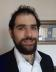# A Regular Question

This weeks perl weekly challenge had a pretty straightforward question: take a string and split it when the characters change. That's a fairly straightforward regex issue:

perl -pE's/(.) \g1*/$& /gx'  Here we match any character (.) once. We capture this in a group. Then, we use backreferencing to match as many more copies of that group as we can. We use the s operator to then replace what we found with itself ($& is the entire match that was found) followed by a space.

Conveniently, the challenge didn't specify what 'splitting' entails, so this is technically all we need to do. However, it gives us an extra empty space at the end of the match. In order to get rid of that (the proverbial second problem introduced by regexes), we have two approaches.

## The RegEx Rabbit Hole

We could tell the regex not to match if it gets to the end of the string. We would do that with a zero width negative lookahead:

(?! $)  Meaning don't match the end of the line. However, this also introduces ANOTHER problem, that now when our regex wants to match something at the end, it will backtrack if it finds more than one of a letter. Meaning if we run our one-liner on 'cc', it will split those two, because it matches the c, then tries to match as many 'c's as possible, then hits the end of the string, and backtracks to only match one c. So to solve that, we can tell the regex engine to be possesive, that when it matches something, it won't give it back. A possesive modifier is a + after the quantifier that you have set. So now, our solution will look like this. perl -pE's/(.) \g1*+ (?!$)/$& /gx'  ## Splitting Up the Issue Alternatively, we could just use split to get rid of the extra space for us. If you don't set a limit on how many fields you want split, then split gets rid of empty trailing fields. So we'll split on the spaces that we put in, and it'll look like this now: perl -pE'split / /, s/(.) \g1*/$& /gx'


This one has the benefit of returning a list, so it can be transplanted into some other code where you'll do more stuff with the list of consecutive letters that you've made.

# Making Friends

The next challenge was to write a script to find the two smallest amicable numbers. These are pairs of numbers whose factors add up to the other number.

SInce this is a regex based post, I'll just show the regex based answer to this challenge.

In order to determine the amicability of two numbers, you need to get the sum of their proper factors. That means every number they're divisible by, except for itself. As it turns out, this is also a pretty easy regex problem. This is a variation of the famous regex for prime numbers:

sub sum_of_proper_divisors {
my $sum = 1; (1 x shift) =~ /^ (..+) \g1+$ (?{ $sum += length$1 }) ./xg;
return $sum }  We first turn the number handed to the function into a string of '1's as long as the number (using the repetition operator 'x'). Then we match a regex against it, looking for a group of at least two characters in a row. We then ask the regex engine to match copies of that group until the end of the string, using a backreference, like we did in the first challenge. Then, after we match the end of the string, we use a regex code block to increment our running $sum by the length of the match. Then we tell the regex to match another character after the end of the string, which it obviously can't, so it backtracks and tries another length for the grouping in our match. It'll continue doing this, breaking down our string of 1's into equal groups, until it can no longer do so. This leaves us with the sum of the factors of our number.

sub has_amicable {
my $start = shift; my$pair = sum_of_proper_divisors($start); #sometimes the sum of the divisors IS the number. But that's not amicable return 0 if$pair == $start; return$start == sum_of_proper_divisors $pair; }  Then we make a function to check if a number has an amicable pair. It finds our potential pair by getting the sum of the divisors of the number passed in. Then it'll return falsy if the "pair" is the same number that we started with, and otherwise return whether or not the$pair's divisors sum back to our starting number.

Then we loop while incrementing our starting number, until we find a pair:

my $num; while (1) { last if has_amicable(++$num);
}

say "$num,${\sum_of_proper_divisors(\$num)} is your pair"


And finally print our 220, 284 is your pair.I do full-stack development with Mojolicious and Vue.